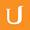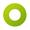## 02ps-02 Symplectic Euler Solution

• 0:00 - 0:05
Let's go over the solution to this problem starting with the definition of the acceleration function.
• 0:05 - 0:07
We know the acceleration due to gravity points downward.
• 0:07 - 0:10
So let's put this factor into two components.
• 0:10 - 0:14
We have one component right here that is parallel to the string of the pendulum
• 0:14 - 0:17
and another component that is perpendicular to this green one.
• 0:17 - 0:22
Now we know that the acceleration in this direction is going to be exactly cancelled out
• 0:22 - 0:25
by the acceleration due to the tension in the rope.
• 0:25 - 0:28
So that means that the acceleration we're looking for is really just this pink component,
• 0:28 - 0:32
which any point along the path is going to be tangent to the trajectory.
• 0:32 - 0:36
Now if we call this angle θ right here, we can figure out the length of this pink component
• 0:36 - 0:42
by just saying that it is equal to length of the resultant vector times the sine of θ.
• 0:42 - 0:47
The θ down here is actually exactly equal to θ in the diagram of the pendulum itself.
• 0:47 - 0:50
So that means to figure out the length of this component,
• 0:50 - 0:53
• 0:53 - 0:58
Since position is just the arc link right here of this imaginary circle,
• 0:58 - 1:02
then the measure of that angle in radiant is going to be equal to the length of the arc
• 1:02 - 1:06
that it corresponds to divided by the radius of that circle.
• 1:06 - 1:13
So that means that in our case, θ is equal to arc length over radius or position over length.
• 1:13 - 1:16
So since θ equals position over length and we want the sine of θ,
• 1:16 - 1:21
we fill in our definition for acceleration as -g or negative
• 1:21 - 1:26
magnitude of the acceleration due to gravity times sine of the position over length.
• 1:26 - 1:29
Okay, moving on towards symplectic Euler function.
• 1:29 - 1:32
We have to fill in this for loop with the input num_initial conditions.
• 1:32 - 1:39
As I said in the InterVideo of the problem, we wanted the initial x to vary from 1.75 to 2.25
• 1:39 - 1:42
and the initial v to vary from -2 to 2
• 1:42 - 1:46
corresponding to the coordinates of every point along that green circle that I'd showed you.
• 1:46 - 1:49
Now a convenient way to make a variable cycle through values that are symmetric
• 1:49 - 1:52
about an equilibrium value is to use sine or cosine.
• 1:52 - 1:54
So we're going to keep that in mind.
• 1:54 - 1:58
Now as you learn from the circular orbit problem of Unit 1, if we consider any point
• 1:58 - 2:04
along the circumference of the circle, then we can define an angle that corresponds to that point.
• 2:04 - 2:06
These are coming from right here as a zero radian mark.
• 2:06 - 2:12
You can write the coordinates of this point then as the radius of the circle times the cosine of the angle.
• 2:12 - 2:15
That's for the horizontal component and for the vertical component,
• 2:15 - 2:18
we get the radius times the sine of the angle.
• 2:18 - 2:23
In the phase based plot that I showed you in the InterVideo, we saw that position lying along
• 2:23 - 2:26
the horizontal axis and velocity lying along the vertical axis.
• 2:26 - 2:32
So we wanted to plot the coordinates of the points on that green circle--the initial condition circle
• 2:32 - 2:36
where the position is going to correspond to cosine and the velocity is going to use sine.
• 2:36 - 2:40
Now I created a variable called phi. You could pick any name you want I guess.
• 2:40 - 2:44
And phi effectively split the circle into 49 segments
• 2:44 - 2:49
by marking out 50 different points along the circumference.
• 2:49 - 2:54
So every time I increases by one, we're going to step to the next point along the circumference.
• 2:54 - 3:00
Since as we saw in the phase base plot, we have a complete circle of green points.
• 3:00 - 3:05
The x values of those green points vary like this with 2 as the middle value
• 3:05 - 3:07
and the v coordinates vary like that.
• 3:07 - 3:10
You noticed that the amplitude in either case corresponds to the
• 3:10 - 3:13
half link of the green shape in that direction.
• 3:13 - 3:18
So actually we have in a phase base plot is an ellipse for that set of initial conditions.
• 3:18 - 3:22
Now that we have our starting additions figured out, we can finally use the symplectic Euler method
• 3:22 - 3:25
to proximate the values with x and v at later sets.
• 3:25 - 3:31
This code right here is just a direct transition pretty much of the equations that I showed you earlier.
• 3:31 - 3:33
Now let's go back to looking at the plot that we get things plugged in
• 3:33 - 3:36
but first let's look at our top two plots.
• 3:36 - 3:40
The horizontal axis in both of them represents time measured in seconds.
• 3:40 - 3:42
The vertical axis in the top one is x measured in meters
• 3:42 - 3:45
and here it is v measured in meters per second.
• 3:45 - 3:52
So you can see that our initial values of x go from 1.5 to 2.25 and v from -2 to 2.
• 3:52 - 3:55
So that corresponds to this green ellipse right here.
• 3:55 - 3:58
• 3:58 - 4:04
phase base is that if we look closely at each one of these ellipses they all have the same area.
• 4:04 - 4:06
Now let's look at the shapes that we have down here in this bottom graph.
• 4:06 - 4:12
If you look closely and do a bit of calculating, you'll notice that all these different color shapes
• 4:12 - 4:16
have the same exact area even though they are well shaped very differently.
• 4:16 - 4:21
This is a great example of how phase base is conserve in the system where energy is conserve.
• 4:21 - 4:24
Now the fact that its conservation principle holds in this diagram
• 4:24 - 4:30
shows how the symplectic Euler method improve upon the accuracy of the forward Euler method.
• 4:30 - 4:34
When the forward Euler method is used, it often result in the energy suddenly increasing.
• 4:34 - 4:38
So it means that the area of each of these shapes down here will get progressively bigger.
• 4:38 - 4:42
Symplectic Euler method, however, confirms much better the equations of motion in physics,
• 4:42 -
It never reflects exactly radical predictions more accurately. Great job with the first problem in Unit 2.
Title:
02ps-02 Symplectic Euler Solution
Team:Udacity
Project:
CS222 - Differential Equations
Duration:
04:48Amara Bot edited English subtitles for 02ps-02 Symplectic Euler SolutionGundega added a translation

• Amara Bot
• Gundega